## C语言的第二次作业

#include<stdio.h>
int main(void)
{
int n,a,i,j,A,B,C,D,E;
scanf("%d",&n);
for(i=0;i<n;i++) scanf("%d",&a[i]);
A=B=C=D=E=0;
for(j=0;j<n;j++){
if(a[j]>=90) A++;
if(a[j]<90&&a[j]>=80) B++;
if(a[j]<80&&a[j]>=70) C++;
if(a[j]<70&&a[j]>=60) D++;
if(a[j]<60) E++;
}
printf("%d %d %d %d %d",A,B,C,D,E);
return 0;
}


1、文字描述

（1）定义n，A，B，C，D，E；

（2）用for语句判断每一个a的值并将其带入A，B，C，D，E中；

（3）输出A，B，C，D，E。

2、流程图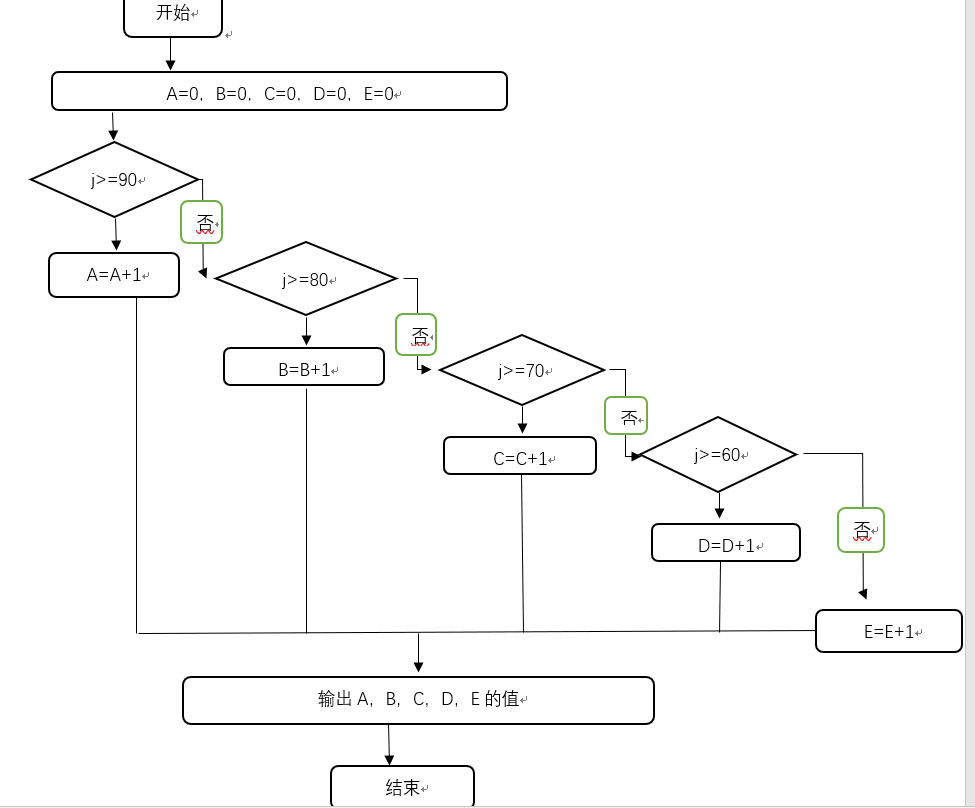三天打鱼两天晒网

#include<stdio.h>
int main(){
int N,c;
scanf("%d",&N);
c=N%5;
if(c==0||c==4){printf("Drying in day %d",N);}
if(c==1||c==2||c==3){printf("Fishing in day %d",N);}
return 0;
}


1、文字描述

（1）定义N，c；

（2）计算c为N除以5的余数；

（3）用if语句判断c的值；

（4）输出语句

2、流程图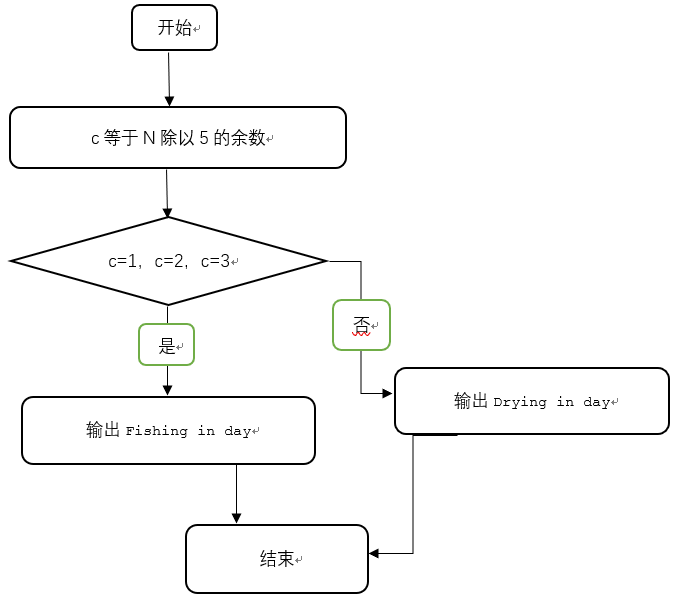错误试例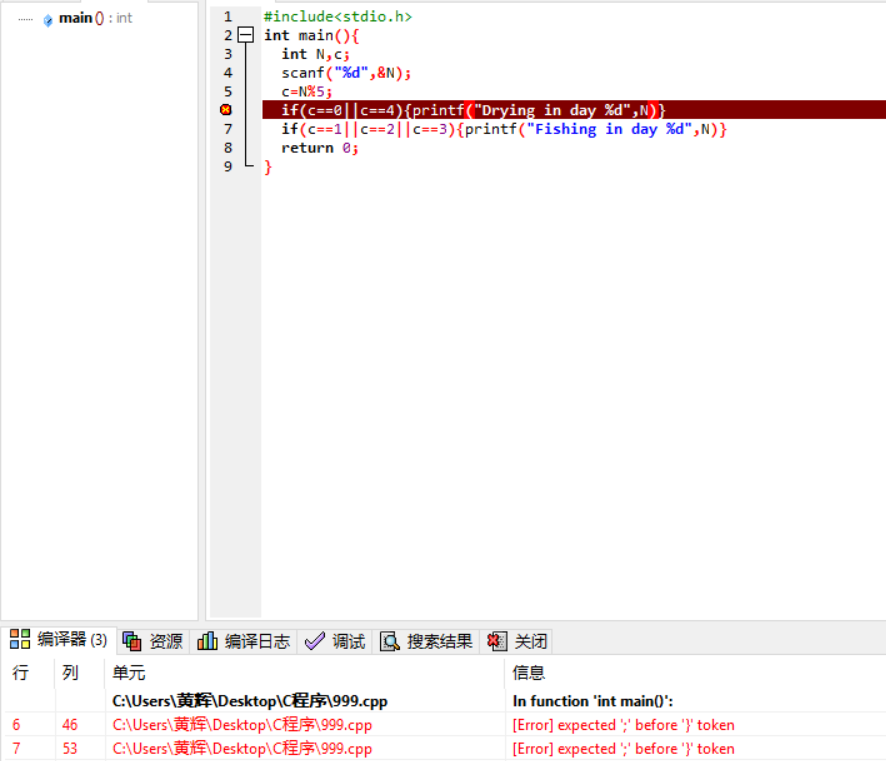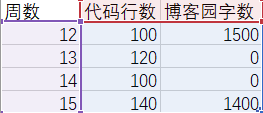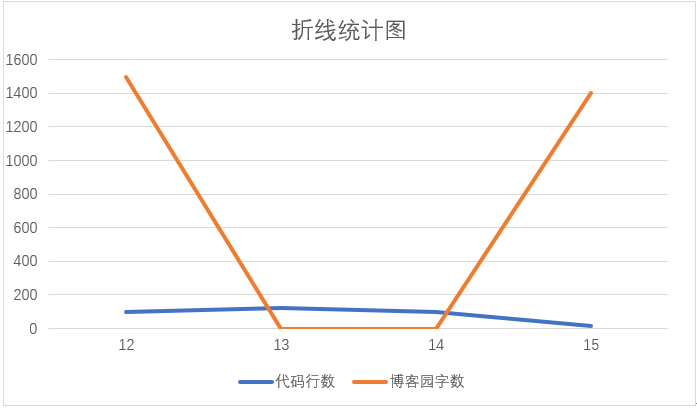posted on 2018-12-08 12:12  七月的长安  阅读(94)  评论(4编辑  收藏  举报